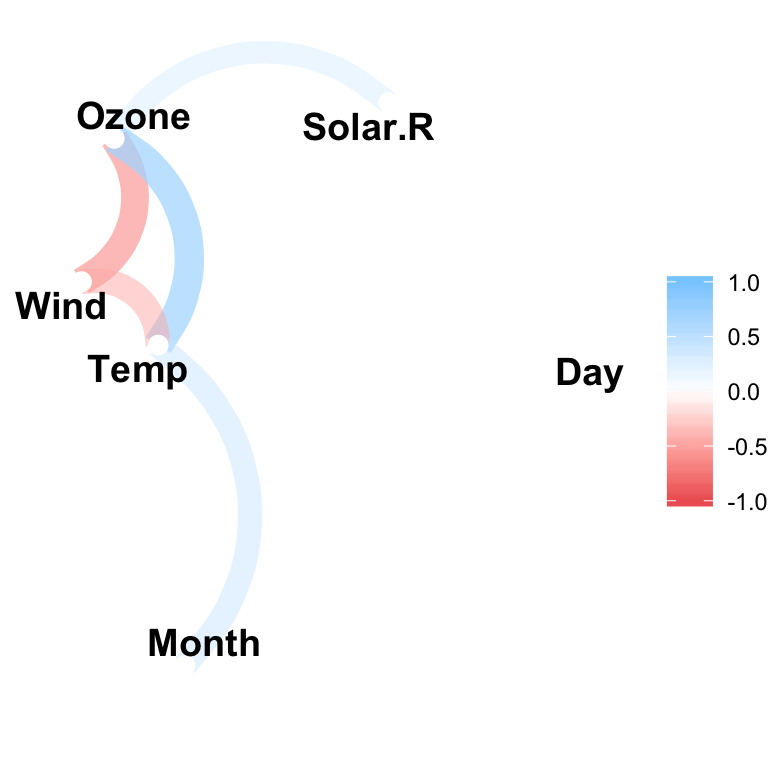# Easily Create a Correlation Network in R using the Corrr Package#### Easily Create a Correlation Network in R using the Corrr Package

This article describes how to plot a correlation network in R using the corrr package.

Related article: Easy Correlation Matrix Analysis in R Using Corrr Package

Contents:

• tidyverse: easy data manipulation and visualization
• corrr: correlation matrix analysis
library(tidyverse)
library(corrr)

## Data

data("airquality")
head(airquality)
##   Ozone Solar.R Wind Temp Month Day
## 1    41     190  7.4   67     5   1
## 2    36     118  8.0   72     5   2
## 3    12     149 12.6   74     5   3
## 4    18     313 11.5   62     5   4
## 5    NA      NA 14.3   56     5   5
## 6    28      NA 14.9   66     5   6

## Compute correlation matrix

res.cor <- correlate(airquality)
res.cor
## # A tibble: 6 x 7
##   rowname    Ozone  Solar.R     Wind    Temp     Month       Day
##   <chr>      <dbl>    <dbl>    <dbl>   <dbl>     <dbl>     <dbl>
## 1 Ozone    NA        0.348   -0.602    0.698   0.165    -0.0132
## 2 Solar.R   0.348   NA       -0.0568   0.276  -0.0753   -0.150
## 3 Wind     -0.602   -0.0568  NA       -0.458  -0.178     0.0272
## 4 Temp      0.698    0.276   -0.458   NA       0.421    -0.131
## 5 Month     0.165   -0.0753  -0.178    0.421  NA        -0.00796
## 6 Day      -0.0132  -0.150    0.0272  -0.131  -0.00796  NA

fashion() the correlations for pleasant viewing:

res.cor %>% fashion()
##   rowname Ozone Solar.R Wind Temp Month  Day
## 1   Ozone           .35 -.60  .70   .16 -.01
## 2 Solar.R   .35         -.06  .28  -.08 -.15
## 3    Wind  -.60    -.06      -.46  -.18  .03
## 4    Temp   .70     .28 -.46        .42 -.13
## 5   Month   .16    -.08 -.18  .42       -.01
## 6     Day  -.01    -.15  .03 -.13  -.01

## Create a correlation network

The R function network_plot() can be used to visualize and explore correlations.

airquality %>% correlate() %>%
network_plot(min_cor = 0.3)The option min_cor indicates the required minimum correlation value for a correlation to be plotted.

Each point reprents a variable. Variable that are highly correlated are clustered together. The positioning of variables is handled by multidimensional scaling of the absolute values of the correlations.

For example, it can be seen from the above plot that the variables Ozone, Wind and Temp are clustering together (which makes sense).

Each path represents a correlation between the two variables that it joins. Blue color represents a positive correlation, and a red color corresponds to a negative correlation.

The width and transparency of the path represent the strength of the correlation (wider and less transparent = stronger correlation).

For example, it can be seen that the positive correlation between Ozone and Temp is stronger than the positive correlation between Ozone and Solar.R.

## Cleaning up the correlation network

We can clean this up by increasing the min_cor, thus plotting fewer correlation paths:

mtcars %>% correlate() %>%
network_plot(min_cor = .7)